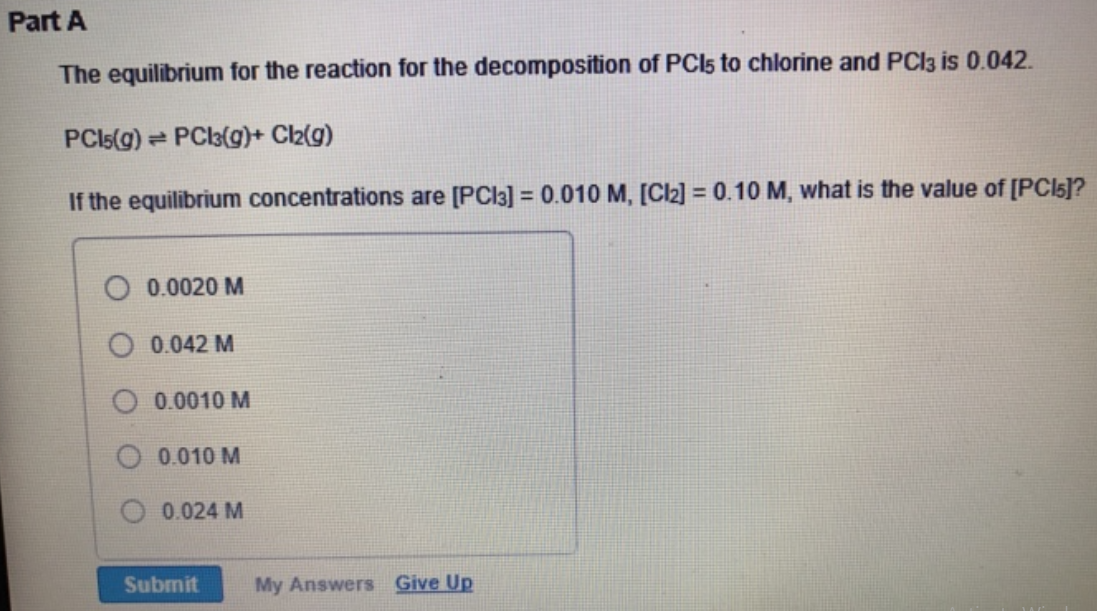Chemistry Practice Problems Equilibrium Expressions Practice Problems Solution: The equilibrium for the reaction for the decomposi...

🤓 Based on our data, we think this question is relevant for Professor Xue's class at GSU.

# Solution: The equilibrium for the reaction for the decomposition of PCl5 to chlorine and PCl3 is 0.042. PCl5(g) ⇌ PCl3(g) + Cl2(g) If the equilibrium concentrations are [PCl3] = 0.010 M, [Cl2] = 0.10 M, what is the value of [PCl5]? (a) 0.0020 M (b) 0.042 M (c) 0.0010 M (d) 0.010 M (e) 0.024 M

###### Problem

The equilibrium for the reaction for the decomposition of PCl5 to chlorine and PCl3 is 0.042.

PCl5(g) ⇌ PCl3(g) + Cl2(g)

If the equilibrium concentrations are [PCl3] = 0.010 M, [Cl2] = 0.10 M, what is the value of [PCl5]?

(a) 0.0020 M

(b) 0.042 M

(c) 0.0010 M

(d) 0.010 M

(e) 0.024 MView Complete Written Solution

Equilibrium Expressions

Equilibrium Expressions

#### Q. Write the equilibrium expression for each of the following dissolution reactions: (a) PbCrO4 (s) ⇌ Pb2+ (aq) + CrO42- (aq)(b) Cs2SO4 (s) ⇌ 2Cs+ (aq) +...

Solved • Tue Jul 17 2018 13:34:41 GMT-0400 (EDT)

Equilibrium Expressions

#### Q. What is the numerical value of Kc for the following reaction if the equilibrium mixture contains 0.025 M N2O4 and 0.20 M NO2? N2O4 (g) ⇌ 2NO2 (g) Expr...

Solved • Mon Jul 16 2018 14:40:38 GMT-0400 (EDT)

Equilibrium Expressions

#### Q. What is the equilibrium expression (Kc) for the following reaction? A(aq) + B(aq) → C(aq)

Solved • Mon Jul 16 2018 13:57:39 GMT-0400 (EDT)

Equilibrium Expressions

#### Q. The elementary reaction 2H2O(g) ⇌ 2H2(g) + O2(g) proceeds at a certain temperature until the partial pressures of H2O, H2, and O2 reach 0.0550 atm, 0....

Solved • Mon Jul 16 2018 13:09:51 GMT-0400 (EDT)